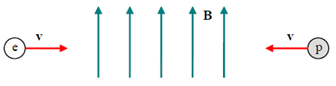# Problem: At a given instant in time, an electron and a proton are moving directly toward each other at the same speed in a uniform magnetic field that is oriented 90° from the velocities of the particles. They experience magnetic forces which are: A. equal in magnitude but perpendicular to each other. B. identical C. equal in magnitude but opposite in direction. D. in opposite directions and differing in magnitude by a factor of 1840. E. in the same direction and differing in magnitude by a factor of 1840.

###### FREE Expert Solution
87% (476 ratings)
###### Problem Details

At a given instant in time, an electron and a proton are moving directly toward each other at the same speed in a uniform magnetic field that is oriented 90° from the velocities of the particles. They experience magnetic forces which are:

A. equal in magnitude but perpendicular to each other.

B. identical

C. equal in magnitude but opposite in direction.

D. in opposite directions and differing in magnitude by a factor of 1840.

E. in the same direction and differing in magnitude by a factor of 1840.